lvy>試卷(2022/08/26)

# 102 年 - 102 國立中山大學_碩士班招生考試_電機系(甲、丙、丁、戊、己組)：工程數學甲#110510

【非選題】
1.

1. (7%) Find the Laurent series representation of a functionwith center at a = j in the domain 1 ＜|z -j |＜ 2, j =.

【非選題】
2.

2. (8%) Evaluate the following integral:where C denotes a counterclockwise simple closed contour |z| = 3.

【非選題】
3.

3. (15%) Compute the Fourier transformof a signum function f(t) defined asEach calculation step is required for obtaining the credit.

【非選題】
4.

4.(15%)下面的問題共有三個子題,只要寫出每個子題的答案即可(不需寫出計算過程),例如: (a) β=1,γ=2。 Let a1 = [1 0 1 α]T,a2=[I β 2 2]T, and a3 = [-2 3 γ -4]T be three vectors in R4, where a, β, and γ are three real parameters, and denote A := [a1 a2 a3].

【題組】

(a) (4%) Suppose a is not a positive integer. Find real β and γsuch that{a1, a2, a3} is a linearly dependent set.

【非選題】
5.【題組】

(b) (5%) Now let a = 2, β = -1,γ = -5, and let x be a nonzero vector in the null space N
(A) of A. Find the value of k to satisty ||x|| 1 + 2||x||∞ + k||x||2 = 0.

【非選題】
6.【題組】

(c) (6%) Now let a = 2, β = -1, γ= -5, and let d denote the distance between vector [1 4 0]T and R(AT), the range space of AT. Compute the value of d.

【非選題】
7.

5.(10%)下面的問題共有二個子題,只要寫出每個子題的答案即可(不需寫出計算過程),例如: (a)θ=30或θ=π/6。
Consider the inner product space C[0, 1] with (f, g) :=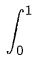f(x)g(x)dc and the nomm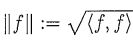. Denote S := span (1, x} as a subspace of C[0, 1].

【題組】

(a) (4%) Compute the angle θ, taken value in [0, π /2), between 1 and x.

【非選題】
8.【題組】(b) (6%) Find a vector u(x) in C[0, 1], so that {1, u(x)} forms an orthonormal basis for S.

【非選題】
9.

6. (20%) Consider the following system of differential equations: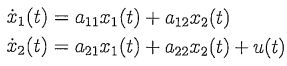where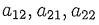are constant coefficients.

【題組】

(a) (5%) Suppose u ≡ 0 and the equations are driven by non-zero initial conditions. Determine the conditions on the coefficientssuch that【非選題】
10.【題組】

(b) (10%) Let the initial conditions be equal to zero. For the values,= -1, andcalculate the response y(t) = 2x1(t) - x2(t). Determine at what time the peak value of y occurs.

【非選題】
11.【題組】

(c) (5%) For the values= -2, and u(t) = sin(t), calculate the steady-state response of y(t)=x1(t)-x2(t).

【非選題】
12.

7. (15%) Consider the region R enclosed by the x-axis, x = I and y = x3, as illustrated below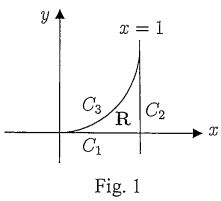【題組】

(a) (3% + 2%) Find the flux of= (1 + y2)j out of R through the two sides C1 (the horizontal segment) and C2 (the vertical segment).

【非選題】
13.【題組】

(b) (10%) Find the flux of= (1 + y2)j out of the third side C3.

【非選題】
14.
8.(10%) Consider the following Lyapunov equation
XA+ATX+Q=0
where A is a (n-dimensional) real square matrix, and X, Q are real symmetric matrices.

【題組】

(a) (5%) Suppose all eigenvalues of A have negative real parts. Show that X =dτ a solution to the Lyapunov equation.

【非選題】
15.【題組】(b) (5%) Suppose Q is positive definite and the Lyapunov equation has a positive definite solution X. Show that all eigenvalues of A have negative real parts.